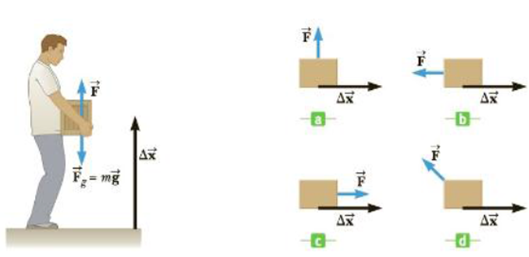Chapter 5.1, Problem 5.1QQ

Chapter
Section
Textbook Problem

In Figure 5.5 (a)-(d), a block moves to the right in the positive x-direction through the displacement Δ x → while under the influence of a force with the same magnitude F → . Which of the following is the correct order of the amount of work done by the force F → , from most positive to most negative? (a) d, c, a, b (b) c, a, b, d (c) c, a, d, bTo determine
The order of the amount of work done by the force from most positive to most negative.

Explanation

The work done on an object using a constant force F causing a linear displacement Δx is,

W=(Fcosθ)d

• d is the magnitude of the displacement
• θ is the angle between the vectors F and Δx

Thus, the maximum work done by the force will be when the angle between the vectors F and Δx is minimum.

In figure c, the angle θ is zero. So, the order of amount of work done will be most positive in figure c.

In figure a, the angle θ is 90°

Still sussing out bartleby?

Check out a sample textbook solution.

See a sample solution

The Solution to Your Study Problems

Bartleby provides explanations to thousands of textbook problems written by our experts, many with advanced degrees!

Get Started